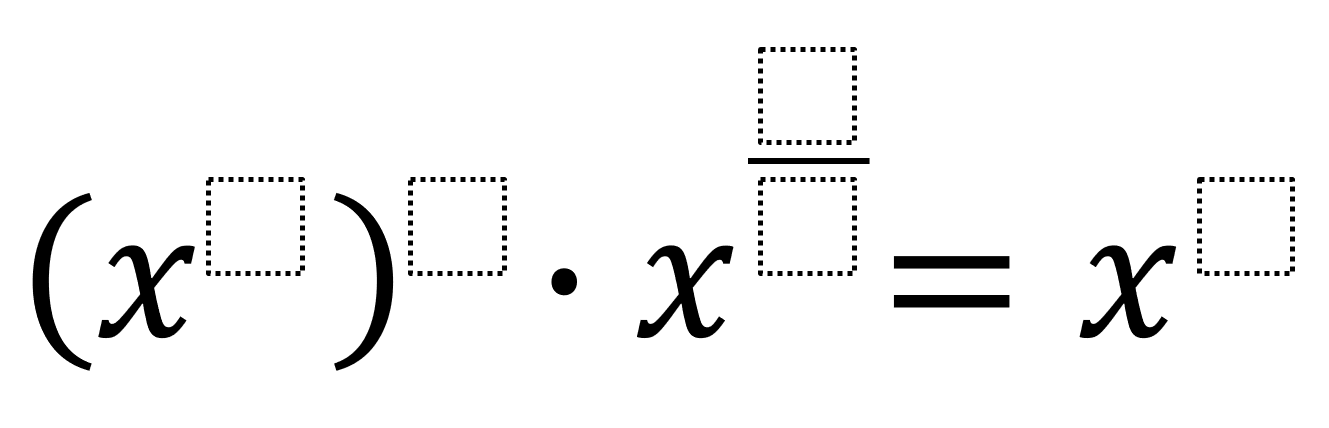# Properties of Exponents 3

Directions: Using the digits 1 to 9 at most one time each, fill in the boxes twice to make an equation. You may reuse all the digits for each equation.### Hint

How do you know when to multiply, divide, subtract, or add the exponents’ value?

There are many possible answers including:
(x^1)^0 · x^(6/3) = x^2
(x^4)^2 · x^(1/5) = x^3

Source: Robert Kaplinsky

## Imaginary Solutions to a Quadratic Equation

Directions: Using the digits 1 to 9 at most one time each, place a digit …

1.I think there is a bit of a mix-up with the second solution. Wouldn’t you add the exponents instead of dividing? So x^8*x^(1/5) = x^(41/5) not x^3

•Absolutely correct.

There’s a problem in the first solution too: 0 was not one of the usable digits.

2.Editing the directions to include the digits 0-9 offers the opportunity to bring to mind what an exponent of 0 means (again…and again and again).